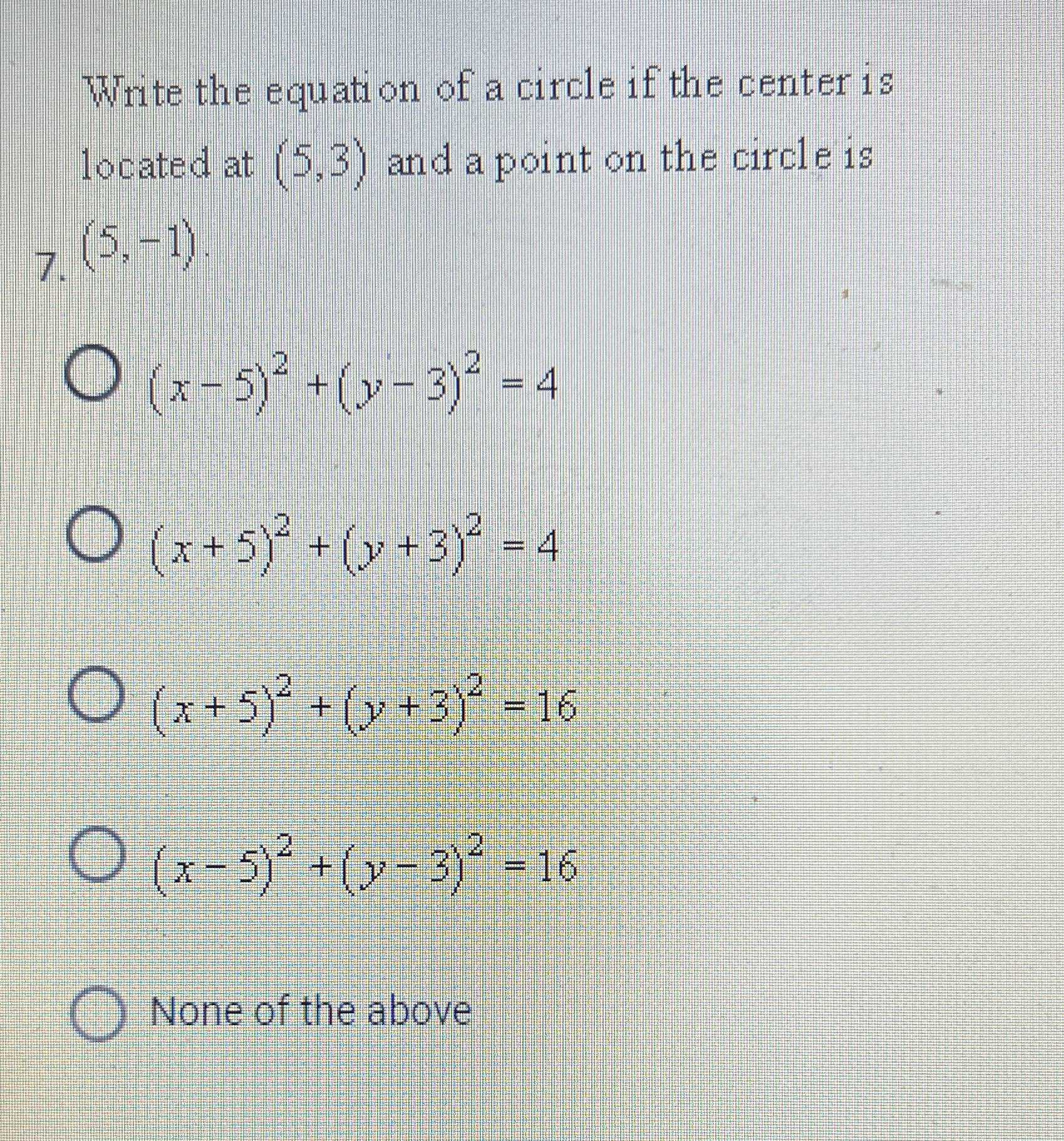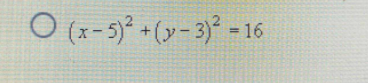### Still have math questions?

Algebra
QuestionWrite the equation of a circle if the center is located at $$( 5,3 )$$ and a point on the circle is $$( 5 , - 1 )$$

$$( x - 5 ) ^ { 2 } + ( y - 3 ) ^ { 2 } = 4$$

$$( x + 5 ) ^ { 2 } + ( y + 3 ) ^ { 2 } = 4$$

$$( x + 5 ) ^ { 2 } + ( y + 3 ) ^ { 2 } = 16$$

$$( x - 5 ) ^ { 2 } + ( y - 3 ) ^ { 2 } = 16$$

None of the above